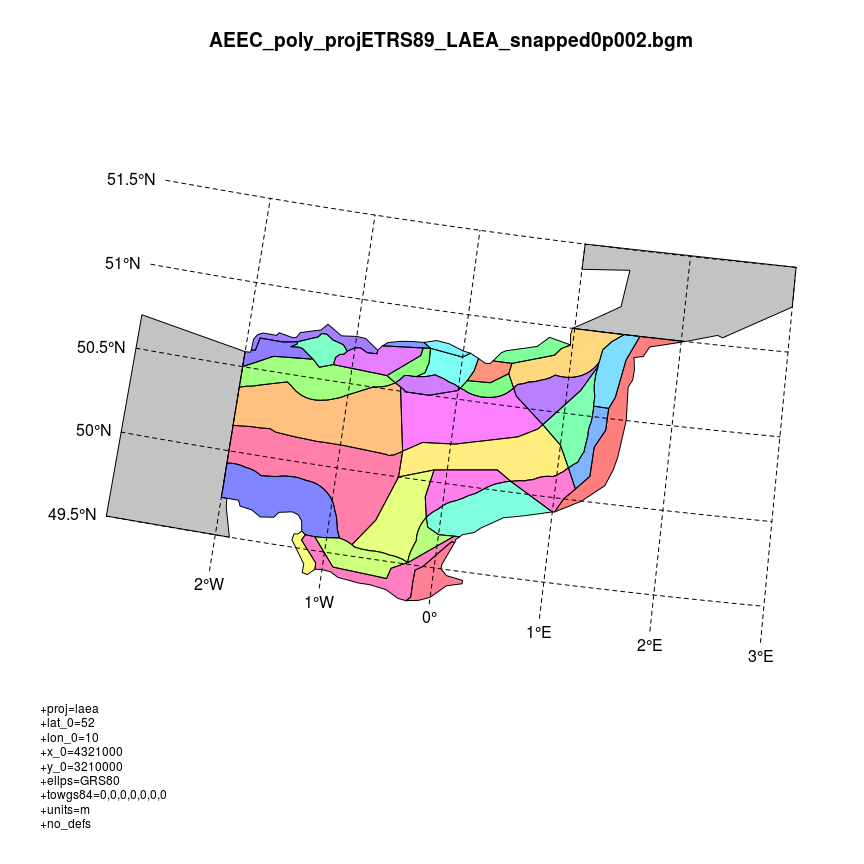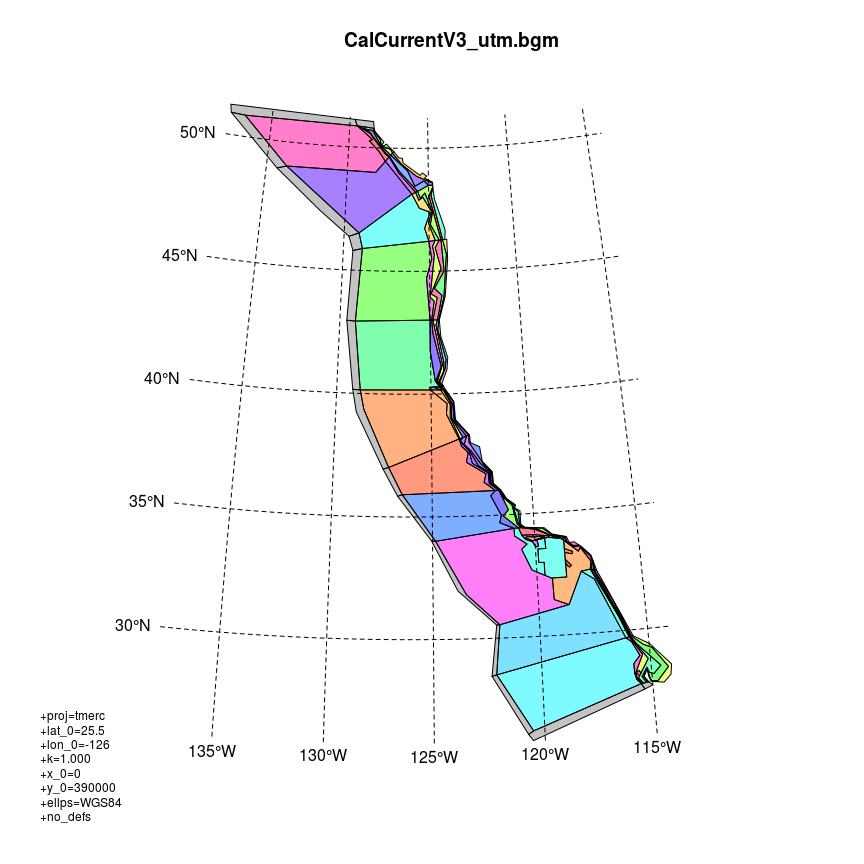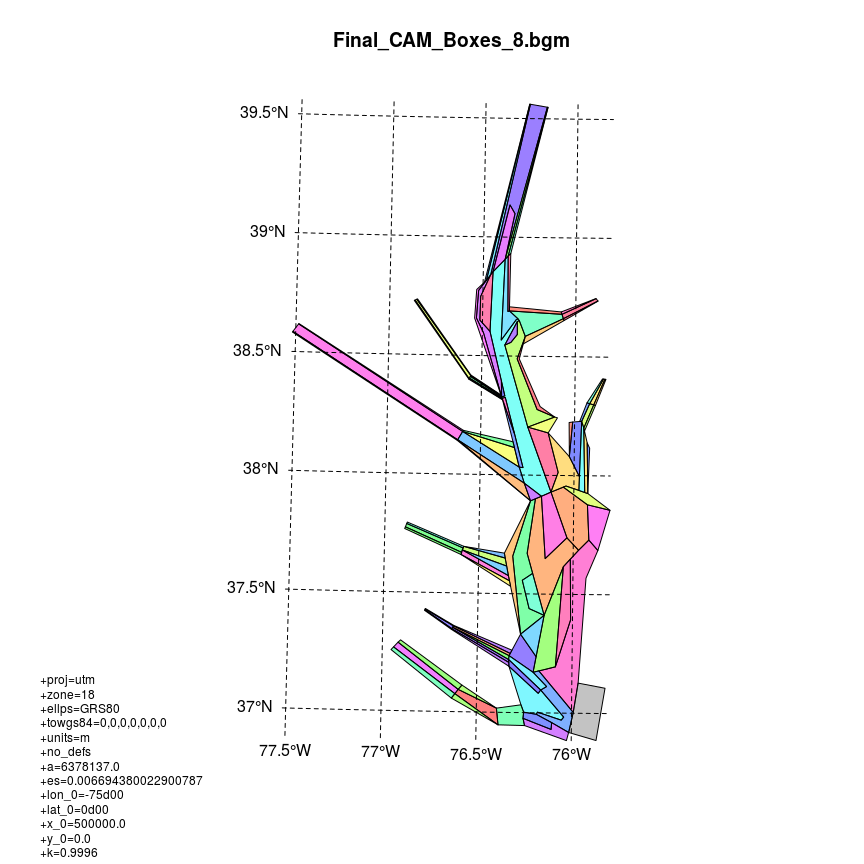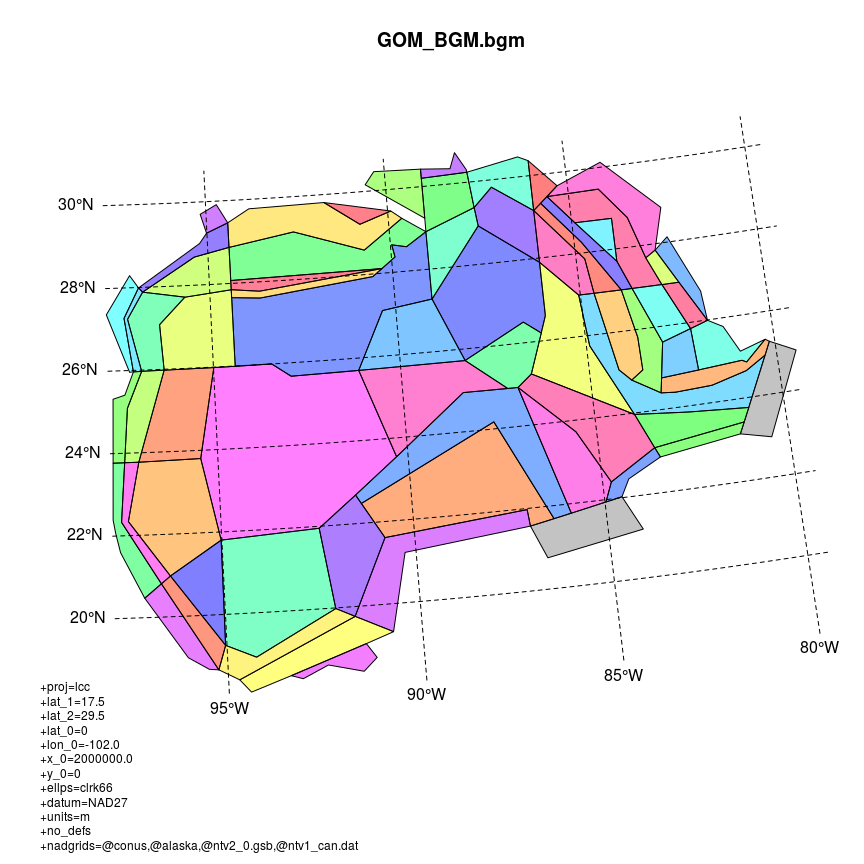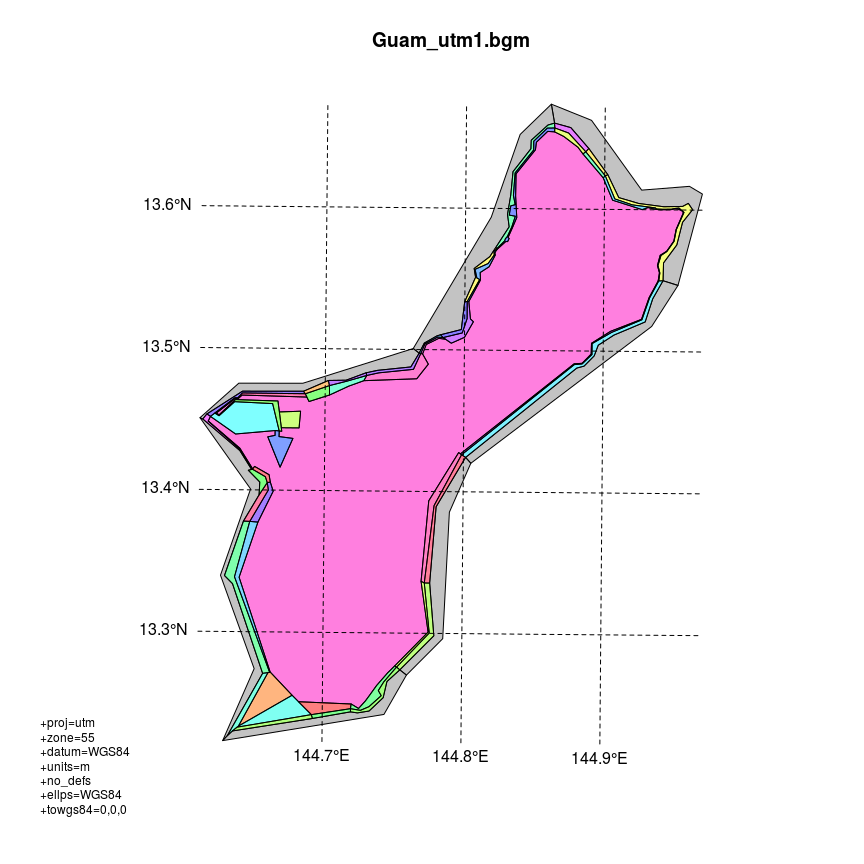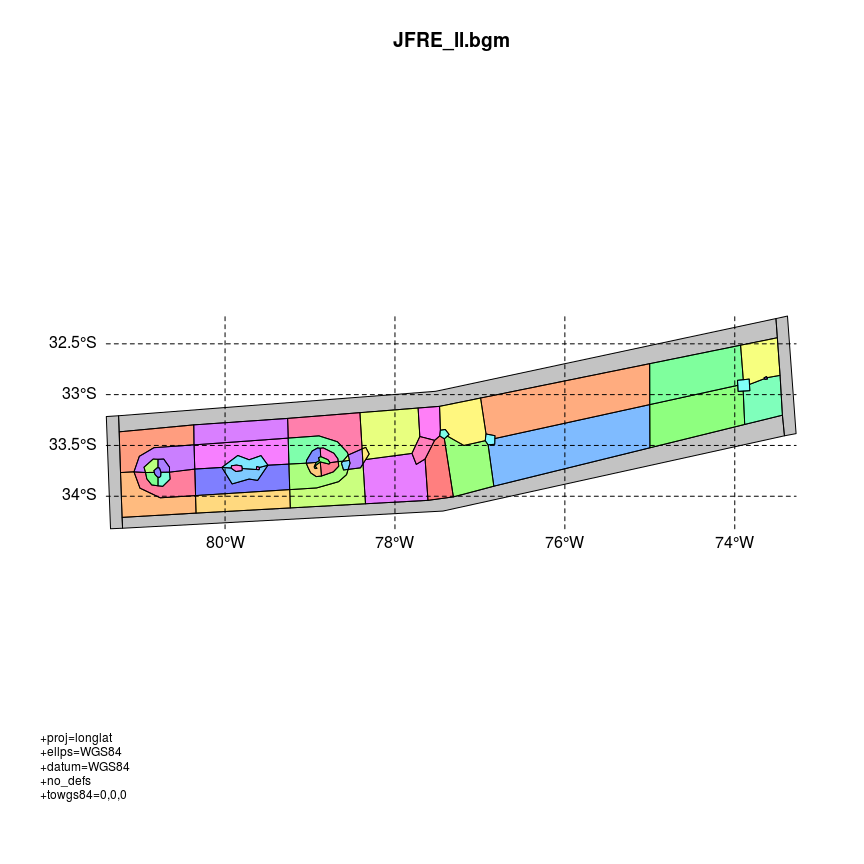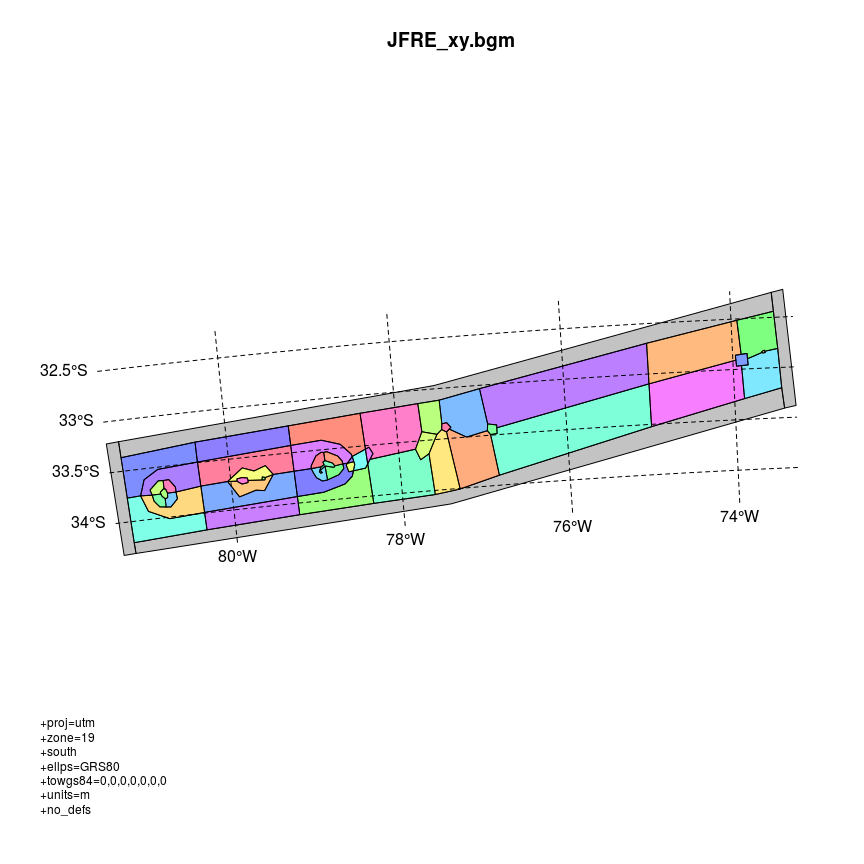# Available BGM examples

The `bgmfiles` package contains several real-world example BGM files.

Obtain every available BGM file and plot in native coordinates, with a graticule.

``````library(rbgm)
library(bgmfiles)

library(rgdal)

## turn +proj into line separated text
breakproj <- function(x) {
paste(strsplit(x, " ")[], collapse = "\n")
}
files <- bgmfiles()
for (i in seq_along(files)) {
bgm <- bgmfile(files[i])
boxes <- boxSpatial(bgm)
plot(boxes, col = ifelse(boxes\$boundary, "#88888880", sample(rainbow(nrow(boxes), alpha = 0.5))))
op <- par(xpd = NA)
llgridlines(boxes)
par(op)
title(basename(files[i]), cex = 0.8)
mtext(breakproj(proj4string(boxes)), cex = 0.75, side = 2, las = 1, adj = 0, line = 2, at = par("usr"), xpd = NA)

}``````